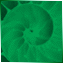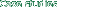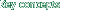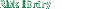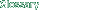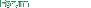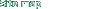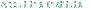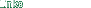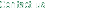Risk Library
 Documents by Author
 Committees at the Bank for International...
 An Internal Model-Based Approach to Mark...I. Common elements of banks' approaches ...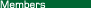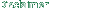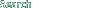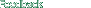### I. Common elements of banks' approaches to risk measurement

 1. The internal models methodology for measuring exposure to market risks is based on the following general conceptual framework. Price and position data arising from the bank's trading activities, together with certain measurement parameters, are entered into a computer model that generates a measure of the bank's market risk exposure, typically expressed in terms of value-at-risk. This measure represents an estimate of the likely maximum amount that could be lost on a bank's portfolio with a certain degree of statistical confidence. 2. The remainder of this section describes the main components of this sequential process as it is typically deployed and serves as background for the discussion of the proposed supervisory framework in the subsequent sections of the paper.3 (a) Inputs 3. The inputs of the measurement system include the following components: Position data, comprising positions arising out of trading activities; Price data on the risk factors that affect the value of the different positions in the portfolio. The risk factors are generally divided into broad categories that include interest rates, exchange rates, equity prices, and commodity prices, with related options volatilities being included in each risk factor category; Measurement parameters, which include the holding period over which the value of the positions can change; the historical time horizon over which risk factor prices are observed (observation period); and a confidence interval for the level of protection judged to be prudent. These measurement parameters are in part judgmental; for example, they may depend on the level of protection that the model seeks to provide, unlike the position and price data which are in principle exogenous. (b) The modelling process 4. Based on the above inputs, an internal valuation model calculates the potential change in the value of each position resulting from specified movements in the relevant underlying risk factors. The changes in value are then aggregated, taking account of historical correlation between the different risk factors to varying degrees - either at the level of an individual portfolio or across trading activities throughout the bank. The movements in risk factors and the historical correlations between them are measured over the observation period chosen by the bank as appropriate for capturing market conditions within its overall strategy. 5. Banks generally use one of two broad methodologies for measuring market risk exposure: variance/covariance matrix methodology and historical simulation. In the case of the variance/covariance methodology, the change in value of the portfolio is calculated by combining the risk factor sensitivities of the individual positions - derived from valuation models - with a variance/covariance matrix based on risk factor volatilities and correlations. The volatilities and correlations of the risk factors are calculated by each bank on the basis of the holding period and the observation period. The confidence level is then used to determine value-at-risk. 6. The historical simulation approach calculates the hypothetical change in value of the current portfolio in the light of actual historical movements in risk factors. This calculation is carried out for each of the defined holding periods over a given historical measurement horizon to arrive at a range of simulated profits and losses. The confidence level is used to determine the value-at-risk. 7. There is also a third, less widely-used approach, the Monte Carlo simulation method, which tests the value of the portfolio under a large sample of randomly chosen combinations of price scenarios, whose probabilities are based on historical experience. This method is particularly useful in measuring the risk in options and other instruments with non-linear price characteristics but is less frequently used to measure the market risk in a broad portfolio of products. (c) Output 8. Each of the measurement methods described produces a final value-at-risk number. Depending on the way the model is constructed, this number may be calculated for individual positions, for different risk factor categories or for exposure to all kinds of market risk. The numbers generated serve as the basis for monitoring exposure levels and exposure limits and at some banks for allocating capital internally across the different business lines of the bank. Footnote: 3. The description in this section is for illustrative purposes and is not intended to define an internal model that is approved for supervisory purposes.